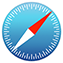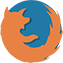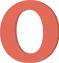Account type Instrument Lot size Currency Leverage Cent Pro ECN Crypto Zero Spread Micro Standard Cent Pro ECN Crypto Zero Spread Micro Standard Cent Pro ECN Crypto Zero Spread Micro Standard Cent Pro ECN Crypto Zero Spread Micro Standard Cent Pro ECN Crypto Zero Spread Micro Standard

Disclaimer: Calculations made in the trading calculator are for informative purposes only and should not be taken as trading advice. Results may vary depending on the data you provide when using the calculator.

## What is margin trading in Forex?

Margin trading — also known as buying on margin — is the practice of borrowing money from your broker to open a larger position than you could with your own capital. In other words, you don’t pay the full price of the trading asset. Instead, you only need to pay a percentage of the position, which is called margin, and the broker lends the rest of the money.

The trading calculator calculates the required margin for each of your trades. You can easily figure out how much money you need to open a position and thus build an effective trading strategy. Here’s how the Forex calculator works.

## How to calculate the margin?

1. Select the Account type (Cent, Micro, Zero Spread, ECN, Crypto, or Standard). For example, let’s choose a Standard account.
2. Select the Instrument you want to trade – a currency pair, Gold, Oil, or another asset. For example, EURUSD.
3. Enter the Lot size (the standard lot in Forex is equal to 100 000 currency units). For example, 0.1 (0.1 lot or 10 000 currency units).
4. Select the Currency in which the margin will be calculated (USD or EUR). For example, USD.
5. Select the Leverage ratio. For example, 1:100.
6. Enter the Ask price or use the automatically inserted market price.
7. Enter the Bid price or use the automatically inserted market price.
8. Click Calculate.

The results: To open a EURUSD position with 0.1 lot, 1:100 leverage, and at the current market price, you will need a margin of 100.91 USD.

The margin calculator can also calculate other important values.

• Contract size is the actual number of units of the selected asset in the calculated position. 1 lot in Forex is equal to 100 000 units of the base currency, while 0.1 lot = 10 000 units (0.1 × 100 000 = 10 000).
• Point value is the amount of money you will earn or lose if the price changes by 1 point.
• Spread is the difference between the price we pay to buy an asset (ask) and the selling price of an asset (bid).
• Swap long is the interest that you either earn or pay per trade for keeping long positions open overnight.
• Swap short is the interest that you either earn or pay per trade for keeping short positions open overnight.

## What is the leverage in Forex?

Leverage is a financial tool that allows a trader to borrow money from a broker in order to open a larger position. It is measured in terms of a ratio between the trader’s own and borrowed funds—1:10, 1:50, 1:100, 1:1000, and so on. Simply put, this is the number of times your funds will be amplified. Leverage trading can multiply your profits on successful trades.

Let’s take a look at how leverage works. For example, if you use 1:100 leverage, every \$1 will turn into \$100. It means you can deposit \$100 and trade like you are using \$10 000. This scheme works the same way with all currencies. Let’s say you have 100 000 USD.

• Leverage of 1:10: 10 000 USD × 10 = 100 000 USD
• Leverage of 1:50: 10 000 USD × 50 = 500 000 USD

## How to choose the leverage?

To choose the right leverage ratio, use the Forex leverage calculator.

1. Select the Account type (Cent, Micro, Zero Spread, ECN, Crypto, or Standard). For example, let’s choose a Standard account.
2. Select the Instrument you want to trade – a currency pair, Gold, Oil, or another asset. For example, EURUSD.
3. Enter the Lot size (the standard lot in Forex is equal to 100 000 currency units). For example, 0.1.
4. Select the Currency in which the margin will be calculated (USD or EUR). For example, USD.
5. Select the Leverage ratio (after clicking the Calculate button, you will know if you made the right guess or if you need to revise the calculation). For example, 1:100.
6. Enter the Ask price or use the automatically inserted market price.
7. Enter the Bid price or use the automatically inserted market price.
8. Click Calculate.

The results: According to the FX leverage calculator, to open a EURUSD position with the selected leverage of 1:100, you will need a margin of 100.91 USD. If you increase your leverage to 1:1000, the required margin will be reduced to 10.09 USD. The rule is simple: the higher the Forex leverage, the lower the margin.

## Why use the Forex leverage?

The most obvious advantage of trading with leverage is to increase capital efficiency and get large potential profit. But there are other benefits as well:

• Leverage lets you make money on even small price changes.
• Leverage allows you to start trading with low initial capital.
• Leverage is an interest-free loan, which means you don’t pay any additional fees when you borrow capital.

Use the FX calculator and try leveraged trading with FBS!

• ## What is the Trader’s calculator?

The Trader’s calculator, or Forex calculator, is a powerful tool that helps you quickly and easily estimate your potential profit and manage risk before opening a trading position.

• ## How to calculate my margin?

To estimate the amount of funds required to open and hold a trading position, specify the required data in the Forex calculator:

• Enter the lot size;
• Select the currency your account is based on;
• Select the leverage rate;
• Enter the ask price and bid price.

Once you click the ‘Calculate’ button, your margin will automatically be shown. This information will help you determine the lot size and leverage so as not to exceed your trading account balance.

• ## Why use the Trader’s calculator?

The Trader’s calculator will help you make the best possible trading decisions before opening positions for the chosen instruments. This is important because different trading parameters can lead to significant changes in spreads, measured in pips, as well as swap long or short, and margin. Therefore, all traders should pre-calculate their trades in the Forex calculator and choose the optimal trading parameters.

## Data collection notice

FBS maintains a record of your data to run this website. By pressing the “Accept” button, you agree to our Privacy policy.

### Callback

A manager will call you shortly.

A manager will call you shortly.

Next callback request for this phone number
will be available in